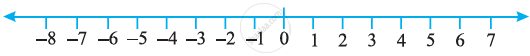Concept for Ordering of Integers:

Let us observe the integers which are represented on the number line.• We know that 7 > 4 and from the number line shown above, we observe that 7 is to the right of 4.
• Similarly, 4 > 0 and 4 is to the right of 0.
• Now, since 0 is to the right of –3 so, 0 > – 3. Again, – 3 is to the right of – 8 so, – 3 > – 8.
• Thus, we see that on a number line the number increases as we move to the right and decreases as we move to the left.
• Therefore, – 3 < – 2, – 2 < – 1, – 1 < 0, 0 < 1, 1 < 2, 2 < 3 so on.
• Hence, the collection of integers can be written as..., –5, –4, – 3, – 2, – 1, 0, 1, 2, 3, 4, 5...

Some important points on Ordering of Integers:

1. Every positive integer is larger than every negative integer.

2. Zero is less than every positive integer.

3. Zero is larger than every negative integer.

4. Zero is neither a negative integer nor a positive integer.

5. Farther a number from zero on the right, larger is its value.

6. Farther a number from zero on the left, smaller is its value.

Comparison of Integers:

(i) - 10 < 10.

(ii) - 10 < 0 < 10.

(iii) - 10,000 < - 1.

If you would like to contribute notes or other learning material, please submit them using the button below.

Shaalaa.com

Concept for Ordering of Integers [00:07:39]
S
0%